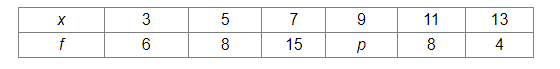# If the mean of the following frequency distribution is 8, find the value of p.

Question:

If the mean of the following frequency distribution is 8, find the value of p.Solution:

We will make the following table:We know:

Mean $=\frac{\sum f_{i} x_{i}}{\sum x_{i}}$

Given:
Mean = 8
Thus, we have:

$8=\frac{303+9 p}{41+p}$

$\Rightarrow 328+8 p=303+9 p$

$\Rightarrow p=25$